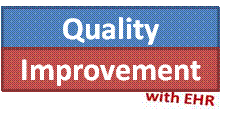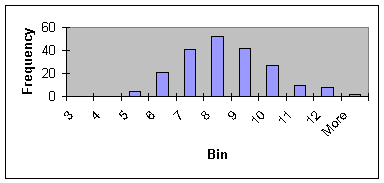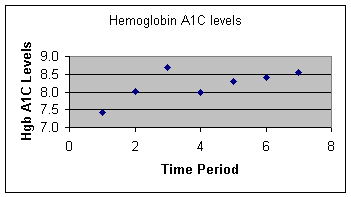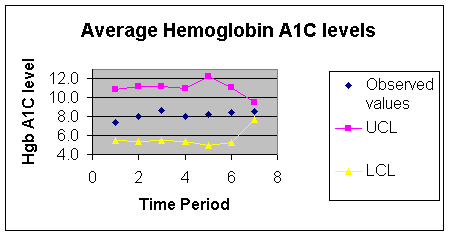# Tutorial on Risk Adjusted X-Bar Charts: Diabetes Control

Farrokh Alemi, Ph.D. & Thomas Sullivan, M.D.

# Purpose of Control charts

Several investigators have reviewed the uses of statistical process control theory in health care.  Applications have ranged from control of excess C-sections, to reduction of medication errors, to prevention of patient falls, and a host of other applications.  In these applications control charts are needed in order to discipline our intuitions about whether changes we have introduced have led to improvements in care outcomes.  Decision makers often mistakenly attribute positive outcomes to their interventions and negative outcomes to random chance or external events.  These perceptions could be wrong.  Control charts enable us to avoid attribution errors regarding our effectiveness.  In addition, control charts tell a chronological and visual story.  They allow us to communicate to others whether the intervention worked.

In the past decade and half, investigators have promoted the use of industrial quality control techniques in health care. But health care and manufacturing differ in one fundamental way.  Input to health care processes is not very much the same.  In many manufacturing processes, such as car making, the inputs, such as a sheet of metal, are always the same.  But in health care, the input unit is usually a patient and the characteristics of those patients may vary tremendously.  Even when patients have the same disease, they differ considerably by severity of the disease and prognosis of their illness.  This difference between health care and industrial processes requires us to modify statistical process control tools so that the tool is appropriate for the health care setting.  This paper shows how patients’ risks can be used to adjust control charts, in particular the X-bar control chart.

Risk adjustments are needed so that we can separate changes in outcomes due to the patient’s prognosis at start of their visit from changes that are due to our intervention in processes of care.  For example, suppose we are trying to reduce c-section rates in our hospital.  We introduce changes in care process and continue collecting data on c-section rates.  At the end of our data collection effort, we are not sure if changes in outcomes are due to the fact that we are now attracting less complicated pregnancies or truly we have reduced our c-section rates.  A risk-adjusted control chart compares observed c-section rates to what could have been expected from the patients’ pregnancy complications.  It allows us to statistically take out differences in outcomes that are due to patients and attributes the remaining changes in outcomes to process of care.

# Focus on X-bar charts

There are many types of control charts available.  Some are designed to trace changes in proportions and probabilities of adverse events (p-charts).   Others are useful in tracking data on one patient (e.g. time-in-between-events chart).  We focus on X-bar charts, a tool widely used for tracking continuous variables (e.g., satisfaction ratings, key clinical findings, health status ratings, etc.) over time.   Eisenstein and Bethea have proposed how to construct risk-adjusted X-bar charts.  Here, we provide a step-by-step approach to the techniques proposed by these authors.

# Data needed

In quality improvement, the purpose of data collection and analysis is not to set blame but to assist improvement efforts.  The purpose is not to prove but to improve.  Often data sets are small and conclusions refer only to process at hand and findings are not intended to be generalized to other environments.  Two data elements are needed for constructing a risk adjusted X-bar chart include:

1. A continuous observed outcome collected over time across a sample of patients.
2. An expected outcome for each patient.

The data needed are available in many circumstances.  Expected outcomes can be based on clinician’s review of patients’ or can be deduced from many commercial and non-commercial severity indices.

# Sample data

In order to help the reader understand risk adjusted control charts, we will present data from a recent analysis we conducted on diabetic patients of an outpatient clinic.  Type 2 Diabetes Mellitus affects millions of Americans each year and, if not controlled, can result in considerable morbidity.   The question of interest to the clinicians was whether they had improved over time in helping their patients control diabetes.  Previous studies have shown that control charts can be constructed on data collected from diabetes patients [vi].  We thought if we look at the average experience of the patients of several providers, we would be able to speak to the skills of the provider in helping their patients control their diabetes.  For our outcome variable, we decided to focus on Hemoglobin A1C levels (HgbA1C) measured in Type 2 Diabetic patients.  Studies have shown that the microvascular complications of retinopathy, nephropathy and neuropathy can be prevented with good control of the blood sugar levels [vii]. Measuring blood glucose gives information for that moment in time but measuring Hemoglobin A1C levels (HgbA1C) gives information on how well controlled the blood glucose levels have been over the preceding 8 weeks.  A HgbA1C level of 7 represents an average blood glucose level of 150 which is considered to show good control of the Diabetes and a higher HgbA1C level represents higher blood glucose levels and thus worse control of the Diabetes.  We reviewed the data on sixty Type 2 Diabetic patients in a Family Practice clinic of five providers for 21 consecutive months and present the data for 2 of those providers..  HgbA1C levels were measured on a quarterly basis to determine if treatments were resulting in good control of the Diabetes.  We will use this data set to demonstrate how to create a risk adjusted X-bar control chart.

# Steps in constructing risk adjusted control charts

There are 9 steps involved in constructing a Risk Adjusted X-bar chart:

1. Check assumptions.
2. Determine the average of all observations in each time period.
3. Create a plot of the averages over time.
4. Calculate and plot expected values using a severity adjustment tool.
5. Calculate the expected average for each time period.
6. Calculate standard deviation of the difference between observed and expected values for each time period.
7. Calculate and plot the control limits.
8. Interpret the findings.
9. Distribute the chart and the findings to the people who “own the process”.

The remainder of this paper will describe each step in detail and show examples using a subset of the larger data collection.

# 1. Check Assumptions

There are 5 assumptions that must be verified before proceeding with the construction of an X-bar control chart.  These include:

1)      Observations are on a continuous interval scale.
2)
Observations are independent of each other.
3)
There are more than 5 observations in each time period.
4)
Observations are normally distributed.
5)
Variances of observations over time are equal.

In the case of our example, the HgbA1C is measured on a continuous interval scale.  There are at least three types of scales.  In a nominal scale, numbers are assigned to objects in order to identify them but the numbers themselves have no meaning.  For example a DRG code 240 assigned to myocardial infarction is a nominal scale.  An ordinal scale is a scale in which the numbers preserve the rank order.  In an ordinal scale, a score of 8 is more than 4 but not necessarily twice more than 4.  An interval scale requires not only that numbers preserve the order but also in correct magnitudes.  Thus, a score of 8 is twice 4.  The difference between two interval scores is meaningful, while the difference between two ordinal score is not.  In our example, HgbA1C measures preserve both order and difference among patients and therefore it is an interval scale.

The second assumption is that each observation is independent.  The measurement of HgbA1C in one patient does not influence the measurement of another patient or of the same patient in another time period.  Therefore, the second assumption regarding independence of observations is met.  Examining correlation among HgbA1C values of same patient at different times can also test assumptions of independence.  Large positive correlations suggest that the assumption of independent observations is violated.

The third assumption focuses on availability of the data in each time period.  It is met because the data set is sufficiently large that there are more than 5 observations in each time period.

The fourth assumption is that observations have a Normal, bell shaped curve.  Chi-squared statistic can be used to test if HgbA1C have a Normal distributionInstead of conducting a chi-squared test, we prefer to visually examine the data by constructing a histogram.  Figure 1 shows the histogram of HgbA1C levels in our data.

Figure 1: Distribution of HgbA1C dataThe distribution is symmetric and peeks in the middle; in short it has a bell shaped curve as expected from a Normal distribution.  Therefore, the fourth assumption that observations have a Normal distribution is not rejected.

The fifth assumption is about equality of variances of observations across time periods.  Analysis of Variance (ANOVA) can be used to test the equality of variance of observations in different time periods.  Here again, we prefer to test the assumption quickly through calculating average ranges.  When the ranges of observations in different time periods are not two or three multiples of each other, then we accept the assumption of equality of variance.  In this case, average ranges differ from low of 5.8 to high of 11.6; all seem to be within the same ballpark.  Therefore, we accept the assumption of equality of variances over time.

# 2. Determine the Average of observations in each time period

Table 1 shows the average of observations for each time period.  In Table 1, each row represents an individual patient and each column is a separate time period.  The observations for each column are summed and then divided by the number of observations for that time period.

Ai = åj=1…ni Aij / ni

In the above formula, Ai is the average of observations for time period i, Aij is the observation ‘j’ in time period “i”, and ni is the number of observations for time period i.

Table 1:  Data for patients of provider 1 over 7 time periods

 Patient Hemoglobin A1C levels 3 months 6 months 9 months 12 months 15 months 18 months 21 months 1 8.8 9.9 10.2 10.5 11.0 2 6.7 9.0 9.0 9.2 8.8 9.2 8.5 3 8.2 7.6 10.8 9.2 8.9 4 6.1 7.7 7.1 9.0 5 9.1 9.6 9.6 8.8 6 11.1 11.5 7 9.1 8.4 7.8 8.5 8 9.7 9.0 11.7 9 5.9 7.6 7.8 6.1 7.0 6.3 10 7.4 7.0 5.7 7.0 11 7.2 7.4 7.0 7.3 8.1 12 8.9 8.1 9.1 9.7 7.9 8.1 13 5.9 7.1 7.8 7.1 7.8 14 9.9 13.5 15 5.1 5.7 5.5 5.0 16 7.4 7.6 17 7.1 6.2 7.0 7.2 7.1 18 8.3 9.2 7.9 9.0 19 7.0 8.6 8.9 8.6 8.2 10.1 10.0 20 6.4 6.9 6.2 7.0 8.4 21 7.0 7.4 Average 7.4 8.0 8.7 8.0 8.3 8.4 8.5

# 3. Create a Plot of averages over time

A plot can help tell a story much more effectively than any statistic.  After calculating the averages for each time period, we create an X-Y plot of averages against time periods.  This is shown in Figure 2.  Note that there seems to be a slight increase over time in the averages and one may be concerned whether in the 6th and 7th time period the patients are showing less control of their diabetes.

Figure 2:  Average HgbA1C for Provider 1 over all 7 time periods# 4.Calculate expected values

The purpose of risk adjustment is to determine if outcomes have improved beyond what can be expected from the patients’ condition.  If they have, then the clinician has provided better or worse than expected care.  If not, changes in patients’ conditions explain the outcomes and quality of care has not changed.  Extensive literature exists regarding factors that increase risk of complication from diabetes.  Many of these, for example smoking, are factors that clinicians can encourage patients to change.  To measure risk, we decided to focus on variables that providers have little control over and that could make the diabetes control more difficult.  We looked at age of onset of diabetes, as data show that patients will have less control on their diabetes over time.  We looked at number of medications, as patients’ ability to control their diabetes maybe hampered by their need to take medication.  For the 60 patients of five providers in our sample data, we regressed HgbA1C levels (averaged across time periods) on two independent variables: number of medications and age of onset of Diabetes.  Table 2 shows the result of the regression.

Table 2:  Regression of HgbA1C on three dependent variables

 Independent variable Coefficient P-value Constant 8.58 0 Age of onset of diabetes -0.03 0.07 Number of medications 0.76 0 Adjusted R2 = 21%

We used the regression equation to predict expected HgbA1C levels for each patient at each time period.  The equation we used is given below:

Hgb A1C level = 8.58 + 0.76*( # meds) + (-.03)*(age of onset)

For each patient at each time period, we calculated a predicted HgbA1C.  For example, for patient one at 3 months (the first time period) the number of medicines was 3 and the age was 65.  Using the above regression equation, we calculated the expected level of 8.2.  The calculated values are our expectation regarding the patients’ ability to control their diabetes.   Table 3 shows the observed and expected values for one provider in two time periods.

Table 3:  Expected and observed values for two time periods for Provider 1

 Patient 3 months 6 months Observed Expected Observed Expected 1 8.8 8.2 9.9 8.2 2 6.7 9.1 9 9.1 3 8.2 9.3 7.6 9.3 4 6.1 9.1 5 9.1 8.4 9.6 8.4 6 11.1 8.8 7 9.1 8.7 8 9 5.9 8.3 7.6 8.3 10 7.4 7.2 7 7.2 11 7.2 7.9 12 8.9 8 8.1 8 13 5.9 7.8 7.1 7.8 14 15 5.1 6.8 16 7.4 7.4 17 7.1 7.3 6.2 7.3 18 8.3 8.6 19 7 9.5 8.6 9.5 20 6.4 7.8 21 7 6.8

# 5. Calculate average of expected values for each time period

To calculate the average of the expected values for a specific time period, Ei , add all expected values in that time period and divide by the number of observations.   If Eij is the expected value for the patient “j” in time period “i”, then the average of these values, Ei, can be calculated as follows:

Ei = åj=1…ni Eij / ni

For the first 3 months in Table 3, the average of expected values is 8.2 and for the second 3-month period the average of the expected values is 8.4.  The following expected averages for subsequent time period for this one provider were 8.3, 8.3, 8.6, 8.2 and 8.6.

# 6. Calculate the standard deviation of the difference between observed and expected values

Suppose Dij shows the difference between observed and expected values for patient “j” in time period “i”, that is:

Dij = Aij - Eij

Furthermore, suppose Di is the average of the differences for the time period “i”, that is:

Di = åj=1…ni  Dij / ni

Then, standard deviation of the differences, Si, is calculated as:

Si = [åj=1,…,ni  (Dij -Di )2 / (ni -1)]0.5

Note that the standard deviation of each time period depends on the number of observation in the time period.  As the number of observations increase, standard deviation decreases and, as we will see shortly, control limits are set tighter and chances for observing points out of the control limits increases.  The calculation of standard deviation using the first two time periods for the 21 patients of 1 provider are shown in Table 4:

Table 4:  Calculation of standard deviation of differences for 21 patients in 2 time periods

 Patient 3 months 6 months Obs-Exp (Diff-Avg Diff)^2 Obs-Exp (Diff-Avg Diff)^2 1 0.6 1.9 1.7 3.92 2 -2.4 2.8 -0.1 0.02 3 -1.1 0.12 -1.7 2.07 4 -3 7.29 5 0.7 2.04 1.2 2.04 6 2.4 6.76 7 0.4 1.27 8 9 -2.4 2.83 -0.7 0.23 10 0.2 0.96 -0.2 0.01 11 -0.7 0.18 12 0.9 2.75 0.1 0.13 13 -1.9 1.42 -0.7 0.24 14 15 -1.7 0.98 16 0.1 0.64 17 -0.2 0.3 -1.1 0.72 18 -0.3 0.2 19 -2.5 3.21 -0.9 0.48 20 -1.4 0.48 21 0.2 0.24 Avg of Diff -0.7 -0.3 Sum of Diff 21.89 24.33 Std Dev of Diff 1.25 1.37

# 7. Calculate and plot the control limits

Control limits are typically set two or three standard deviations away from the expected values.  When the limits are set at two standard deviations away from the expected values, then 95% of the values are expected to fall within the limits.  There is 5% chance to erroneously conclude that the system is out of control, when in fact it is not.  When the limits are set three standard deviations away from the expected values, 99.7% of the data are expected to fall within limits.  Then the chance of making an erroneous conclusion drops to 0.3%.  Tighter limits are chosen when the cost of making an erroneous conclusion is high.  Wider limits are chosen when it is important to detect changes in the process, even if occasionally (5% of time) one makes an erroneous conclusion. The upper control limit for time period “i”, shown as UCLi, is calculated as:

UCLi = Ei + t * Si

In this equation, “t” is a constant that depends on the number of cases used in the time period and the confidence interval adopted.  Table 5 gives the “t” values for various sample sizes and confidence intervals.

Table 5: t-values for various sample sizes and confidence intervals

 Sample Size 5 10 15 20 25 95% confidence 2.57 2.22 2.13 2.09 2.06 99% confidence 4.03 3.17 2.95 2.84 2.79 For sample sizes not provided, estimate proportional to nearest values provided.

Thus, for the 10 cases in time period one, the UCL1 is calculated to be:

UCL1 = E1 + t * S1

UCL1 = 8.6 + 2.22 * 1.26

The lower control limit for time period “i”, shown as LCLi, is calculated as:

LCLi = Ei - t * Si

Thus, for the 10 cases in the first time period, the LCL1 is calculated to be:

LCL1 = E1 - t * S1

LCL1 = 8.6 - 2.22 * 1.26 = 5.81

Sometimes lower control limits are negative numbers.  If it is not possible to observe negative number, as often is the case, the lower control limit is set to zero.  In this case, the lower control limit is a positive number and therefore we do not need to change it.  When control limits have been calculated for all time periods, then these limits are plotted.  The following Figure 3 shows the observed values plotted against seven time periods for cases of one provider.  Lower and upper control lines are superimposed on these figures to enable quick interpretation of the findings.  Similar figures can be constructed for other providers, thus making it possible to compare provider’s performance despite differences in their case mix.

Figure 3: Control Chart for Provider 1# 8. Interpret findings

Any points that fall within control limits are variations that can be expected by chance alone.  Points outside the two limits indicate observations that are not within our expectations.  For example, in the diabetes data, a point above the control limit indicates a time period in which patients’ HgbA1C is worst than expected.  Any point below the LCL indicates a time period in which HgbA1C are better than expected.  In our data, no points were outside control limits.  Therefore, over time both clinicians had maintained the HgbA1C of their patients at the same level.  Interventions to encourage patients to lower their HgbA1C had not paid off beyond what could have been expected from patients’ conditions.

# 9.Distribute the chart and the findings

In the final step, the chart and the findings are distributed to the improvement team.  In providing feedback about the data, make sure that you follow these principles:

1. Always give your assumption and visually display the testing of the assumptions.
2. Explain how expected values were calculated.  Make sure that you clearly state that if expectations are changed conclusion will change
3. Provide the risk adjusted control chart.
4. Write a brief interpretation of the findings, including whether the process has changed.  Annotate the control chart if special causes variation occurs, for example if “new improvement started”, “Dr. A joined the practice,” “New medication was started.”
5. Distribute the analysis to both the improvement team, and with their approval, to other process owners who might be interested in the team’s deliberation.

This page is part of the b> course on Quality / Process Improvement, the lecture on X-bar charts.  Copyright © 1996 Farrokh Alemi, Ph.D. Created on Sunday, October 06, 1996 4:20:30 PM